# Texas Go Math Grade 6 Lesson 13.4 Answer Key Multiplication and Division Inequalities with Rational Numbers

Refer to our Texas Go Math Grade 6 Answer Key Pdf to score good marks in the exams. Test yourself by practicing the problems from Texas Go Math Grade 6 Lesson 13.4 Answer Key Multiplication and Division Inequalities with Rational Numbers.

## Texas Go Math Grade 6 Lesson 13.4 Answer Key Multiplication and Division Inequalities with Rational Numbers

Investigating Inequality Symbols

You have seen that multiplying or dividing both sides of an inequality by the same positive number results in an equivalent inequality. How does multiplying or dividing both sides by the same negative number affect an inequality?
A. Complete the tables.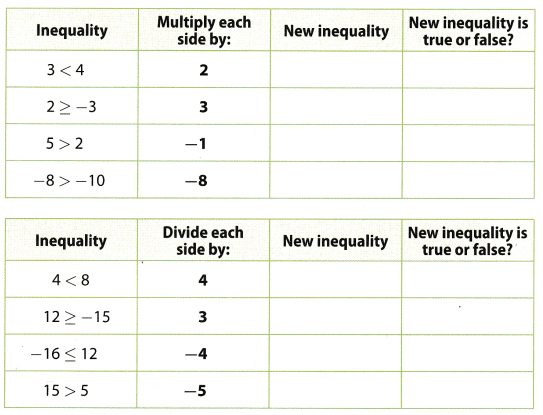B. What do you notice when you multiply or divide both sides of an inequality by the same negative number?
C. How could you make each of the multiplication and division inequalities that were not true into true statements?

Solve each inequality. Graph and check the solution.

Question 1.
-10y < 60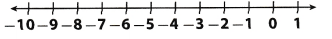First step is to divide both sides by 10. 10 is negative number, so, we need to reserve the inequality symbol for the statement to still be true. So, we have the following:
$$\frac{-10x}{10}$$ > $$\frac{60}{-10}$$
x > -6
Now we will graph the solution:Next step is to check the solution using substitution. We will substitute 10 for x in original inequality:
-10 ∙ 10 < 60
-100 < 60 So, conclusion is the statement is true. x > -6

Question 2.
7 ≥ –$$\frac{t}{6}$$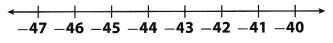First step is to multiply both sides by 6. 6 is negative number, so, we need to reserve the inequality symbol the statement to still be true So, we have the following:
(-6) ∙ 7 ≤ (-$$\frac{t}{6}$$)(-6)
-42 ≤ t
Now we will graph the solution: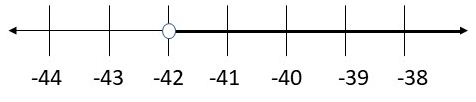Next step is to check the soLution using substitution. We will substitute -40 for t in original inequality:
7 ≥ –$$\frac{-40}{6}$$
7 ≥ 6.67
So, conclusion is the statement is true.
t ≥ -42

Question 3.
Every month, $35 is withdrawn from Tom’s savings account to pay for his gym membership. He has enough savings to withdraw no more than$315. For how many months can Tony pay for his gym membership?
Let x be number of months Tony can pay for his gym membership. Using alt given informations, we get the following inequality we need to solve:
-35x ≥ -315
First step is to divide both sides by -35. -35 is negative number, so, we need to reserve the inequality symbol for the statement to still be true. So, we have the following:
$$\frac{-35 x}{-35} \leq \frac{-315}{-35}$$
x ≤ 9
So, Tony has enough money to pay membership at most for 9 months.
Now, we will check our result by substituting a value smaller than 9 in the original inequality:
-35 ∙ 5 ≥ -315
-175 ≥ -315
So, the statement is true.
x ≤ 9

Solve each inequality. Graph and check the solution. (Explore Activity and Example 1)

Question 1.
-7x ≥ 21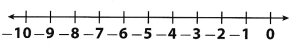First step is to divide both sides by -7. -7 is negative number, so, we need to reserve the inequality symbol, for the statement to still be true So, we have the following:
$$\frac{-7 z}{-7} \leq \frac{21}{-7}$$
z ≤ -3
Now we will, graph the solution: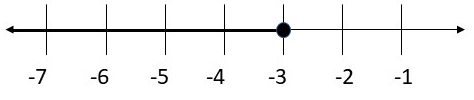Next step is to check the soLution using substitution. We will substitute -5 for z in original inequality:
-7 ∙ (-5) ≥ 21
35 ≥ 21
So, conclusion is the statement is true.
z ≤ -3

Question 2.
–$$\frac{t}{4}$$ > 5First step is to multiply both sides by -4. -4 is negative number, so, we need to reserve the inequality symbol for the statement to still be true So, we have the following:
(-4) (-$$\frac{t}{4}$$) < 5(-4)
t < – 20
Now we will graph the solution:Next step is to check the solution using substitution. We will substitute -40 for t in original inequality:
–$$\frac{-40}{4}$$ > 5
10 > 5
So, conclusion is the statement is true.
t < -20

Question 3.
11x < -66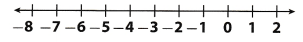First step is to divide both sides by 11. So, we have the following:
$$\frac{11 x}{11}<-\frac{66}{11}$$
x < -6
Now we will graph the solution: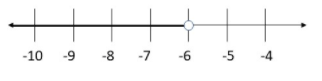Next step is to check the solution using substitution. We will substitute 8 for x in original inequality:
11 ∙ (-8) < – 66
-88 < – 66
So, conclusion is the statement is true.
x < -6

Question 4.
–$$\frac{t}{10}$$ > 5First step is to multiply both sides by -10. -10 is negative number, so, we need to reserve the inequality symbol for the statement to still be true. So, we have the following:
(-10) (-$$\frac{t}{10}$$) < 5(-10)
t < -50
Now we will graph the solution:Next step is to check the solution using substitution. We will substitute 70 for t in original inequality:
–$$\frac{-70}{10}$$ > 5
7 > 5
So, conclusion is the statement is true.
t < -50

Question 5.
For a scientific experiment, a physicist must make sure that the temperature of a metal does not get colder than -80 °C. The metal begins the experiment at 0 °C and is cooled at a steady rate of -4 °C per hour. How long can the experiment run? (Example 2)
a. Let t represent time in hours. Write an inequality. Use the fact that the rate of change in temperature times the number of seconds equals the final temperature.
If t represent time in hours, using all informations we have, get the following inequality: -4t > -80.
1 hour has 60 seconds, so, Let s represent time in seconds Rate of change in temperature is -4°C per hour but rate of change in temperature per second would be –$$\frac{4}{60}$$° C, or, –$$\frac{1}{15}$$° C.
So, we have following equation:
–$$\frac{1}{15}$$s = -80

b. Solve the inequality in part a. How long will it take the physicist to change the temperature of the metal?
In order to solve inequality from part (a), we need to divide both sides by -4. -4 is negative number, so, we need to reverse the inequality symbol for the statement to still be true.
So, we have the following:
$$\frac{-4 t}{-4}<\frac{-80}{-4}$$
t < 20

c. The physicist has to repeat the experiment if the metal gets cooler than -80°C. How many hours would the physicist have to cool the metal for this to happen?
Again, let t represent time in hours, using all informations in this part, we get the following inequality we need to solve:
-4t < -80
In order solve it, we need to divide both sides by 4. In order to solve inequality from part (a), we need to divide both sides by -4. -4 is negative number, so, we need to reverse the inequality symbol for the statement to still be true. So, we have the following: $$\frac{-4 t}{-4}>\frac{-80}{-4}$$
t > 20
Conclusion is that the physicist would have more than 20 hours for the metal to be cooler than -80°C.

Essential Question Check-In

Question 6.
Suppose you are solving an inequality. Under what circumstances do you reverse the inequality symbol?
We must reserve the inequality symbol for the inequality to still be true, if we multiply or divide both sides of inequality by the same negative number.

Solve each inequality. Graph and check your solution.

Question 7.
–$$\frac{q}{7}$$ ≥ -1First step is to multiply both sides by -7. -7 is negative number, so, we need to reverse the inequality symbol for the statement to still be true So, we have the following:
(7)(-$$\frac{q}{7}$$) ≤ (-1)(-7)
q ≤ 7
Now, we will graph the solution: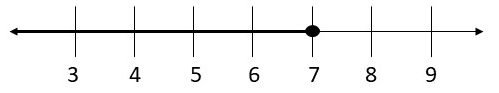Next step is to check the solution using substituting. We wilt substitute 5 for q into the original inequality:
–$$\frac{5}{7}$$ ≥ -1
-0.71 ≥ 1
So, the statement is true.
q ≤ 7

Question 8.
-12x < 60First step is to divide both sides by -12. -12 is negative, number, so, we need to reverse the inequality symbol for the statement to still be true. So, we have the following:
$$\frac{-12 x}{-12}>\frac{60}{-12}$$
x > -12
Now, we will graph the solution: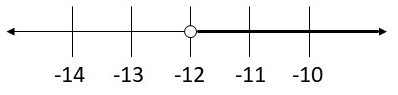Next step is to check the solution using substituting. We will substitute 0 for x into the original inequality:
-12 ∙ 0 < 60
0 < 60 So, the statement is true. x > -12

Question 9.
0.5 ≤ $$\frac{y}{8}$$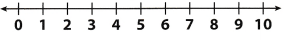First step is to multiply both sides by 8. 8 is positive number. so,we do not need to reverse the inequality symbol.
We have the following:
8 ∙ 0.5 ≤ ($$\frac{y}{8}$$)8
4 ≤ y
Now, we will graph the solution:Next step is to check the solution using substituting. We will substitute 16 for y into the original inequality:
0.5 ≤ $$\frac{16}{8}$$
0.5 ≤ 2
So, the statement is true.
y ≥ 4

Question 10.
36 < -6r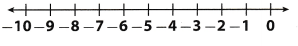First step is to divide both sides by -6. -6 is negative number number, so, we need to reverse the inequality symbol for the statement to still be true. So, we have the following:
$$\frac{36}{-6}>\frac{-6 r}{-6}$$
-6 > r
Now, we will graph the solution: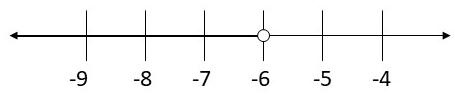Next step is to check the solution using substituting. We will substitute -9 for x into the original inequality:
36 < (-6) (-9)
36 < 54
So, the statement is true
r < -6 Question 11. -12 > 2x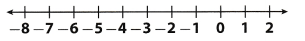First step is to divide both sides by 2. 2 is negative number, so, we need to reverse the inequality symbol for the statement to still be true. So, we have the following:
$$\frac{-12}{2}>\frac{2 x}{2}$$
-6 > x
Now, we will graph the solution:Next step is to check the solution using substituting. We will substitute 9 for x into the original inequality
-12 > 2(-9)
-12 > -18
So, the statement is true.
x < -6

Question 12.
–$$\frac{x}{6}$$ ≤ -0.5
First step is to multiply both sides by -6. -6 is negative number, so, we need to reverse the inequality symbol for the statement to still be true. So, we have the following:
(-6) (-$$\frac{x}{6}$$) ≥ (-0.5) (-6)
x ≥ 3
Now, we will graph the solution:Next step is to check the solution using substituting. We will substitute 12 for x into the original inequality:
–$$\frac{12}{6}$$ ≤ -0.5
-2 ≤ -0.5
So, the statement is true.
x ≥ 3

Question 13.
Multistep Parav is playing a game in which he flips a counter that can land on either a -6 or a 6. He adds the point values of all the flips to find his total score. To win, he needs to get a score less than -48.
a. Assuming Parav only gets 6s when he flips the counter, how many times does he have to flip the counter?
According to all informations given in this part, we get following inequality we need to solve:
-6s > -48
In order to solve it, we need to divide both sides by -6. -6 is negative number, so, we need to reverse the inequality symbol for the statement to still be true. So, we have the following:
$$\frac{-6 s}{-6}<\frac{-48}{-6}$$
s < 8 Conclusion is that Parav need to flip the counter less than 8 times.

b. Suppose Parav flips the counter and gets five 6s and twelve -6s when he plays the game. Does he win? Explain. Answer:
Here, because Parav flips the counter and gets five 6s, s = 5, so, here we actually have 6 ∙ 5 Next, he has twelve 6s, so, s = 12 and we have -6 ∙ 12. We get the following inequality which we need to check: 6 ∙ 5 – 6 ∙ 12 > -48
30 – 72 > -48
-42 > -48
We got that this statement is true, so, conclusion is that in this case, Parav wins.

Question 14.
A veterinarian tells Max that his cat should lose no more than 30 ounces. The veterinarian suggests that the cat should lose 7 ounces or less per week. What is the shortest time in weeks and days it would take Max’s cat to lose the 30 ounces?
Let x represent the time in weeks and days it would take Max’s cat to lose the 30 ounces. According to all informations, we have the following inequality:
-7x > -30
In order to solve it, we need to divide both sides by -7. -7 is negative number, so, we need to reverse the inequality symbol for the statement to still be true. So, we have the following:
$$\frac{-7 x}{-7}<\frac{-30}{-7}$$
x < $$\frac{30}{7}$$ = 4$$\frac{2}{7}$$
So, it would take no more than 4 weeks and 2 days to Max’s cat to lose required weight.

Question 15.
The elevation of an underwater cave is -120 feet relative to sea level. A submarine descends to the cave. The submarine’s rate of change in elevation is no greater than -12 feet per second. How long will it take to reach the cave?
Let x represent time in seconds it will take to a submarine to reach the cave. According to all informations in this task, we have the following inequality:
-12x ≤ -120
In order to solve it, we need to divide both sides by 12. 12 is negative number so, we need to reverse the inequality symbol for the statement to still be true. So, we have the following:
$$\frac{-12 x}{-12} \geq \frac{-120}{-12}$$
x ≥ 10
Conclusion is that it will take at least 10 seconds to a submarine to reach the cave.

Question 16.
The temperature of a freezer is never greater than -2°C. Yesterday the temperature was -10 °C, but it increased at a steady rate of 1.5 °C per hour. How long in hours and minutes did the temperature increase inside the freezer?
Let x represent time in hours and minutes for which the temperature increased inside the freezer. Because the temperature of freezer is never greater than -2°C and yesterdays temperature was -10°C, the difference between those two temperatures is 8°C So, using all informations ¡n this task, we have the following inequality:
-1.5x < -8 In order to solve it, we need to divide t by -1.5. -1.5 is a negative number, so we need to reverse the inequality symbol for the statement to still be true. So, we have the following: $$\frac{-1.5 x}{-1.5}>\frac{-8}{-1.5}$$
x > 5.33
Conclusion is that it will take more than 5.33, or about 5 hours, to temperature increase inside the freezer.

Question 17.
Explain the Error A student’s solution to the inequality -6x > 42 was x > -7. What error did the student make in the solution? What is the correct answer?
Here, student divided both sides by -6, but -6 is negative number and in that case he needed to reverse the inequality symbol for the statement to still be true. So, the right way to solve this inequality would be the following:
$$\frac{-6 x}{-6 x}<\frac{42}{-6}$$
x < -7
So, the set of solutions is x < – 7.

Solve each inequality.

Question 18.
18 ≤ -2x
In order to solve this inequality, we need to divide both sides by -2. -2 is negative number, so, we need to reverse the inequality symbol for the statement to still be true. So, we have the following:
$$\frac{18}{-2} \geq \frac{-2 x}{-2}$$
-9 ≥ x
Now, we will check the solution substituting some value for x into the original inequality from previous set of solutions. We will substitute -18 for x and get:
18 ≤ 2 ∙ (-18)
18 ≤ 36
So, the statement is true.
x ≥ -9

Question 19.
–$$\frac{x}{7}$$ ≤ -23
In order to solve this inequality, we need to multiply both sides by -7. -7 is negative number so, we need to reverse the inequality symbol for the statement to stilL be true. So, we have the following:
(-7)(-$$\frac{x}{7}$$) ≥ (-23) (-7)
x ≥ 161
Now, we will check the solution substituting some value for x into the original inequality from previous set of solutions. We will substitute 200 for x and get:
–$$\frac{200}{7}$$ ≤ -23
-28.57 ≤ -23
So, the statement is true.
x ≥ 161

Question 20.
–$$\frac{x}{8}$$ < –$$\frac{1}{2}$$ Answer: In order to solve this inequality, we need to multiply both sides by -8. -8 is negative number, so, we need to reverse the inequality symbol for the statement to still be true. So, we have the following: (-8) (-$$\frac{x}{8}$$) > (-$$\frac{1}{2}$$) (-8)
x > 4
Now, we will click the solution substituting some value for x into the original inequality from previous set of solutions. We will substitute 16 for x and get:
–$$\frac{16}{8}$$ < –$$\frac{1}{2}$$
-2 < –$$\frac{1}{2}$$ So, the statement is true. x > 4

Question 21.
0.4 < -x Answer: In order to solve this inequality, we need to multiply both sides by -1. -1 is negative number, so, we need to reverse the inequality symbol for the statement to still be true So, we have the following: (-1)(-0.4) > (-x)(-1)
-0.4 > x
Now we will check our solution substituting some value from the set of solutions we got in original inequation. We will substitute -1 for x into the original equation:
0.4 < -(-1)
0.4 < 1 So, the statement is true. -0.4 > x

Question 22.
4x < $$\frac{1}{5}$$
First step is to divide both sides by 4. 4 is positive number, so,we do not need to reverse the inequality symbol. We have the following: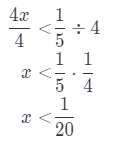Now we will check our solution substituting some value from the set of solutions we got. in original inequation. We will substitute 0 for x into the original equation:
4 ∙ 0 < $$\frac{1}{5}$$
0 < $$\frac{1}{5}$$
So, the statement is true.

Question 23.
–$$\frac{x}{0.8}$$ ≤ -30
In order to solve this inequality, we need to multiply both sides by 0.8. 0.8 is negative number, so, we need to reverse the inequality symbol for the statement to still be true. So, we have the following:
(0.8) (-$$\frac{x}{0.8}$$) ≥ (-30)(-0.8)
x ≥ 24
Now we will check our solution substituting some value from the set of solutions we got in original inequation. We will substitute 30 for x into the original equation:
–$$\frac{30}{0.8}$$ ≤ -30
-37.5 < -30
So, the statement is true.
x ≥ 24

Question 24.
Use the order of operations to simplify the left side of the inequality below. What values of x make the inequality a true statement? –$$\frac{1}{2}$$(32 + 7)x > 32
First we need to calculate vaLue of expression in brackets and then multipLy numbers on the left side So, we have
the following:
–$$\frac{1}{2}$$(9 + 7) x > 32
–$$\frac{1}{2}$$ ∙ 16x > 32
-8x > 32
In order to solve this equation is to divide both sides by -8. -8 is negative number, so, we need to reverse the inequality symbol for the statement to still be true. So, we have the following:
$$\frac{-8x}{8}$$ < $$\frac{32}{-8}$$
x < -4 Now we will check our solution substituting some value from the set of solutions we got. in original inequation. We will substitute -6 for x into the original equation: (-8) (-6)> 32
48 > 32
So, the statement is true x < -4.

H.O.T. Focus on Higher Order Thinking

Question 25.
Counterexamples John says that if one side of an inequality is 0, you don’t have to reverse the inequality symbol when you multiply or divide both sides by a negative number. Find an inequality that you can use to disprove John’s statement. Explain your thinking.
For example, if we have an inequality -4x < 0, in order to solve it, we need to divide both sides by 4.
According to John’s statement we do not need to reverse the inequality symbol, so, let’s try to solve it on that way.
We have the following:
$$\frac{-4 x}{-4}<\frac{0}{-4}$$
x < 0
Now, we will check our solution substituting some value from the set of solutions we got in original inequation. We will substitute -2 for x into the original inequality.
(-4) (-2) < 0
8 < 0
So, we can notice that inequality is not true. Conclusion is that we need to reverse the inequality symbol anyway.

Question 26.
Communicate Mathematical Thinking Van thinks that the answer to -3x < 12 is x < -4. How would you convince him that his answer is incorrect?